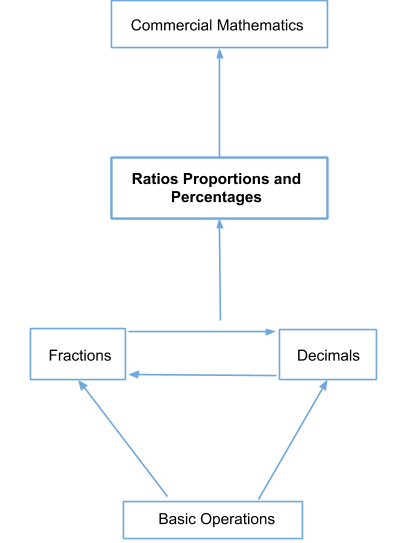# Ratio, Proportions and Percentages

Go back to  'Maths'

The world of mathematics of numbers is vast and varied. When your child was introduced to numbers, their main focus was on understanding value and place. Then the focus shifted to internalising operations such as addition, subtraction, multiplication and division. From there they journeyed through the lands of factors, multiples and prime numbers. Now, they have arrived here, in the domain of fractions, decimals, ratios, proportions and percentages.

## The Foundational Nature of Ratio, Proportions and Percentages## The Big Idea: What are Ratios, Proportions and Percentages?

These three topics are a natural extension of the concept of fractions and decimals. Therefore, make sure you check out those pages and thoroughly understand them before attempting to start on ratios, proportions and percentages.

## Sub Topics

Here are a few links that will take you through the journey that every Cuemath students undertakes in the pursuit of understanding Ratios and Proportion, Percentages and Unitary Method along with practice worksheets: